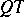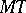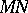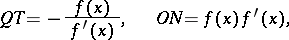# Subtangent and subnormal

The directed segmentsandwhich are the projections on the-axis of the segments of the tangent lineand the normalto a certain curve at a point(see Fig.).Figure: s091040a

If the curve is the graph of a function, the values of the subtangent and subnormal are equal torespectively, whereis the abscissa of the point. If the curve is given parametrically bythenwhereis the value of the parameter defining the pointon the curve.